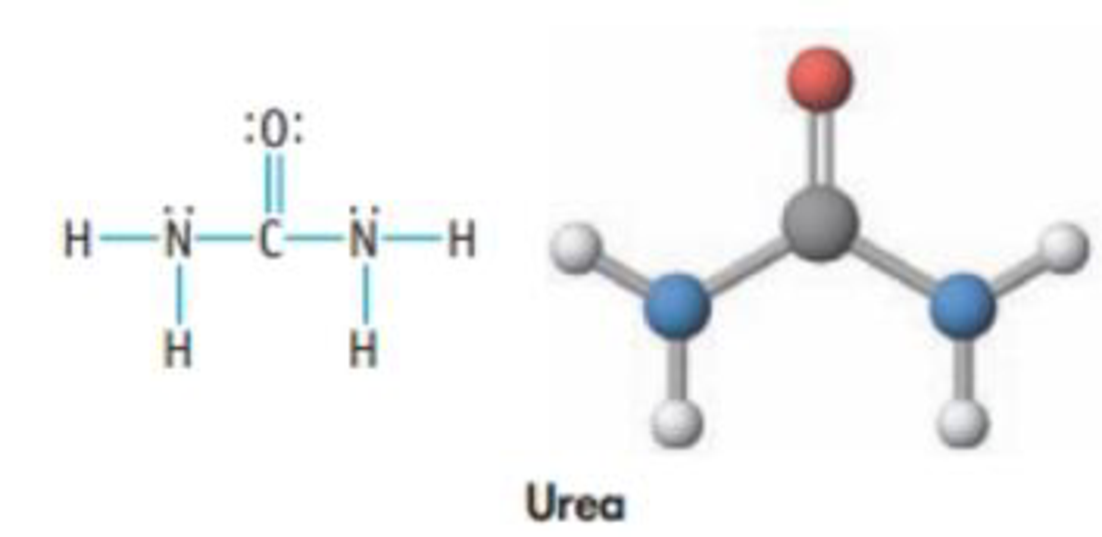Chapter 8, Problem 30PS

Chapter
Section
Textbook Problem

Urea, (NH2)2CO, is used in plastics and fertilizers. It is also the primary nitrogen-containing substance excreted by humans. (a) Which bonds in the molecule are polar, and which are nonpolar? (b) Which is the most polar bond in the molecule? Which atom is the negative end of the bond dipole?(a)

Interpretation Introduction

Interpretation:

The bonds in urea which are polar and non-polar have to be determined.

Concept introduction:

Polar covalent bond involves an unequal sharing of electrons between two bonded atoms; it creates a partial separation of charge and a dipole moment.

Covalent bonds are polar, if there is a difference in electronegativity between the bonded atoms.

Explanation

The given compound is urea

The polar bonds and the non-polar bonds in urea have to be determined.

The electronegativity of atoms in a bond will be the deciding factor to predict whether the bond is polar or non-polar.

Electronegativities of the atoms in urea are given below:

Oxygen3.5Carbon2.5Nitrogen3.0Hydrogen2

(b)

Interpretation Introduction

Interpretation:

The most polar bond in urea molecule and the atom in the negative end of the bond dipole have to be identified.

Concept introduction:

Polar covalent bond involves an unequal sharing of electrons between two bonded atoms; it creates a partial separation of charge and a dipole moment.

Covalent bonds are polar, if there is a difference in electronegativity between the bonded atoms

Still sussing out bartleby?

Check out a sample textbook solution.

See a sample solution

The Solution to Your Study Problems

Bartleby provides explanations to thousands of textbook problems written by our experts, many with advanced degrees!

Get Started

Find more solutions based on key concepts